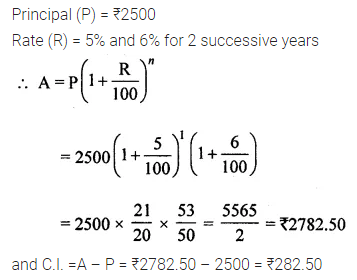# ML Aggarwal Class 8 Solutions for ICSE Maths Chapter 8 Simple and Compound Interest Check Your Progress

## ML Aggarwal Class 8 Solutions for ICSE Maths Chapter 8 Simple and Compound Interest Check Your Progress

Question 1.
Find the amount and the compound interest on ₹5000 for 2 years at 6% per annum interest payable yearly.
Solution:Question 2.
Find the amount and the compounded interest on ₹7400 for 1 year at 5% per annum, interest payable half-yearly.
Solution:Question 3.
Find the difference between C.I. and S.l. on a sum of ₹5000 for 2 years at 8% per annum payable yearly.
Solution: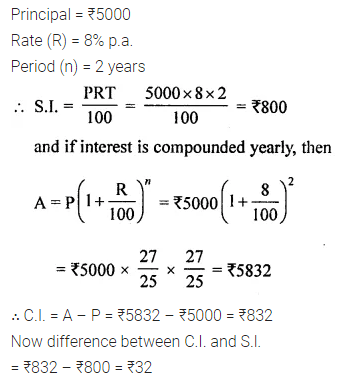Question 4.
Find the amount and compound interest on ₹10000 for $$1 \frac{1}{2}$$ years at 10% per annum, compounded half yearly. Would this interest be more than the interest he would get if it was compounded annually?
Solution: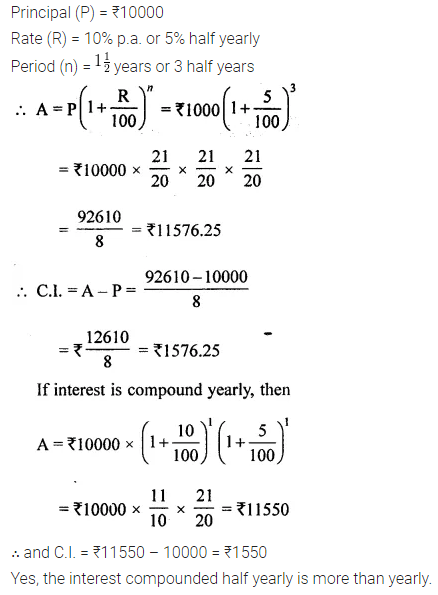Question 5.
What sum invested for $$1 \frac{1}{2}$$ years compounded half-yearly at the rate of 4% p.a. will amount to ₹ 132651?
Solution: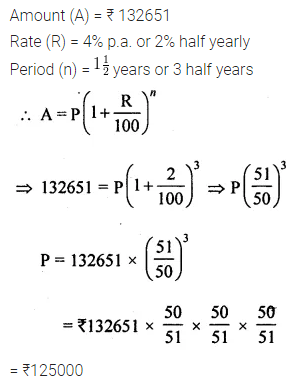Question 6.
Find the time (in years) in which ₹ 12500 will produce ₹3246.40 as compound interest at 8% per annum, compounded annually.
Solution: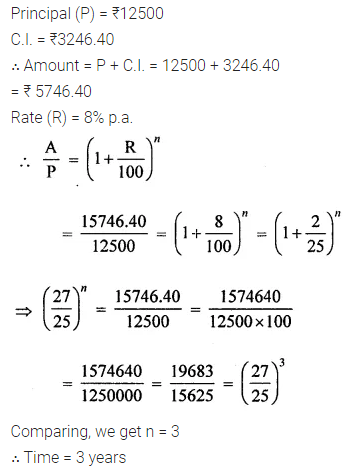Question 7.
Find the amount and compound interest on ₹2500 in 2 years if the rate are 5% and 6% for the successive years.
Solution: